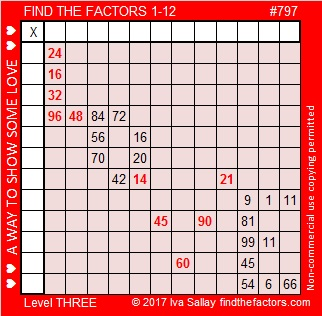# 797 and Mathematical Ways to Love

Mathwithbaddrawings.com has some thoughtful and entertaining Ways to Tell a Mathematician that you love them.

Artful Maths wrote a post that includes beautiful mathematical origami valentines and a “string art” cardioid that is made with a pencil instead of string.

This puzzle could be another mathematical way to show some love:Print the puzzles or type the solution on this excel file: 12-factors-795-799

Here are a few facts about the number 797:

797 is a palindrome in three bases:

• 797 BASE 10 because 7(100) + 9(10) + 7(1) = 797
• 565 BASE 12 because 5(144) + 6(12) + 5(1) = 797
• 494 BASE 13 because 4(169) + 9(13) + 4(1) = 797

But there’s one more palindromic fact about the number 797: It is the sum of two square numbers that are also palindromes!

• 797 = 121 + 676. Note that 11² = 121 and 26² = 676.

Since it is the sum of two squares, 797 will also be the hypotenuse a Pythagorean triple:

• 555 – 572 – 797 calculated from 26² – 11², 2(26)(11), 26² + 11².

797 is the sum of three squares seven different ways:

• 28² + 3² + 2² = 797
• 27² + 8² + 2² = 797
• 24² + 14² + 5² = 797
• 24² + 11² + 10² = 797
• 22² + 13² + 12² = 797
• 21² + 16² + 10² = 797
• 20² + 19² + 6² = 797

797 is also the sum of the 15 prime numbers from 23 to 83:

• 23 + 29 + 31 + 37+ 41 + 43 + 47 + 53 + 59 + 61 + 67 + 71 + 73 + 79 + 83 = 797

Here is the most basic information about the number 797:

• 797 is a prime number.
• Prime factorization: 797 is prime.
• The exponent of prime number 797 is 1. Adding 1 to that exponent we get (1 + 1) = 2. Therefore 797 has exactly 2 factors.
• Factors of 797: 1, 797
• Factor pairs: 797 = 1 x 797
• 797 has no square factors that allow its square root to be simplified. √797 ≈ 28.231188.

How do we know that 797 is a prime number? If 797 were not a prime number, then it would be divisible by at least one prime number less than or equal to √797 ≈ 28.2. Since 797 cannot be divided evenly by 2, 3, 5, 7, 11, 13, 17, 19, or 23, we know that 797 is a prime number.

Here’s another way we know that 797 is a prime number: Since  its last two digits divided by 4 leave a remainder of 1, and 26² + 11² = 797 with 26 and 11 having no common prime factors, 797 will be prime unless it is divisible by a prime number Pythagorean triple hypotenuse less than or equal to √797 ≈ 28.2. Since 797 is not divisible by 5, 13, or 17, we know that 797 is a prime number.This site uses Akismet to reduce spam. Learn how your comment data is processed.